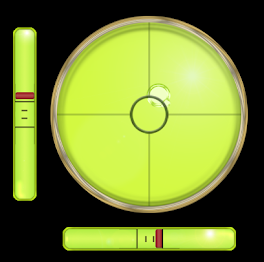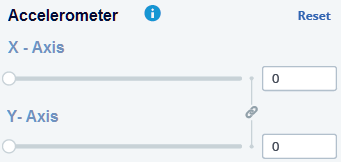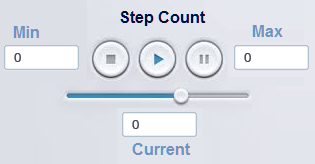# New Facer Editor UI

Really cool. I’ve been thinking about this for a while and have some great ideas as well. Just never put them to paper.

1 Like

I’d like to see more options in the standalone beta creator. The web version can stay the dumbed down one, but a more advanced version could be available as a desktop app.

1 Like

I see a lot of potentials in the ideas here.

Trust the users of the program, trust the developers of the program.

They know best, what is needed… and where it could lead to in future…

I think a lot of companies wish to have such creative, active and innovative human ressources potential…

Here are visionaires…

I at not as experienced as some of you guys but the frustrations of not being able to zoom or have folders I am sure goes from new people to experienced ones. I guess I am used to Illustrator. This UI is great without feeling like I am being bombarded with too much. I agree about the web portal simple or at least a way to turn on beginner or advance mode. Since I will never be able to reach Pro level, hopefully these features won’t just be for them. Can we get some text on a curve love if possible? Another thought coming from a math newbie, maybe animated idea of what the math does. There are some great tutorials but seeing the what the code does helps to know what I am looking for, or at least a link to the well written tutorials. When you don’t know what you are supposed to put in the search bar can steer some new people away.
Facer, this user has something worth doing.

IT SEEMS ME A GREAT JOB!

I think the priority would be to group layers and block them!
in my case it would be great since I work with an immense amount of layers!

3 Likes

Yes. Grouping of elements/layers was my wish as well. Some of my watch faces have about 50 layers.

5 Likes

Grouping of layers would be great as well as a zoom. I think the stand alone creator should have an option to save to hard drive and then sync to facer servers later. This way you can create offline if your not in an area with internet.

3 Likes

more intuitive interface, I like it

How about grouping of layers? Did someone mention grouping of layers yet? I’d like some grouping of layers. Let’s group layers.

I’d actually like some variables too. With programming being a hobby, I’m used to setting variables so that I can use them in different places without having to update in multiple places if I change them. It would be nice if I use a duplicate of a formula to able to write it once in one place and then only update there.

Example:
\$(#DWFSS#-(60*(floor(#Ds#/10))))>=55?((60*(floor(#Ds#/10)))-660)+((#DWFSS#-(60*(floor(#Ds#/10))))12):(60(floor(#Ds#/10)))\$

Variable @T@

``````(60*(floor(#Ds#/10)))
``````

Variable @M@

``````60
``````

Variable @C@

``````55
``````

Variable @S@

``````(@M@/(@M@-@C@))
``````

Variable @O@

``````@S@*@C@
``````

Actual formula

``````\$(#DWFSS#-@T@)>=@C@?(@T@-@O@)+((#DWFSS#-@T@)*@S@):@T@\$
``````

Now I only need to change one value at one place to get the rest of the values I need without having to calculate and update it manually. This would allow for some pretty complex formulas that would still be simple to follow and change.

1 Like

@faustland

I’ve been thinking about this lately due to the difficulty in testing. I wish there was a Master Testing Page. Here are some of the ideas:

Accelerometer Tester: something to move the watch face in each direction. You could move the box or center point on a circle and also have boxes to enter a specific coordinate.Heart Rate/Step Count/Temp: A simple player style UI. You could enter a min and max, then “play” the heart rate and see it increase over time. You could also enter a static numberSome other things that could be tested:

• Random Functions
• Weather Condition
2 Likes

one other thought. I would add the ability to increase the size of the canvas, also add in a ruler and the ability to drag down guide lines:

3 Likes

More ideas:

More Text Options

• Vertical type
• Warp
2 Likes

I completely agree about canvas size, it can be frustrating when I want to zoom in and can’t. I agree on guidelines/rulers to help with placement. Especially when Facer resizes (wish I knew what ratio it does so). Thank you for backing up my suggestion of more type options. Did anyone mention Clipping Masks?

1 Like

It is essentially the same as what I just wrote here:

And consider this example: (which is far TOO complex to debug and use)

from: (second posting in) [SOLVED] How To Tag Moon Phase Today Rotation

`(((((floor(365.25*(#DM#<2?(#Dy#-#Dy#):#Dy#)))+(floor(30.6001*((#DM#<=2)?((#DM#+1)+12):(#DM#+1))))+#Dd#+1720995)+2-((#Dd#+31*(#DM#+12*#Dy#))>=(15+31*(10+12*1582))?(floor(0.01*((#DM#<=2)?(#Dy#-#Dy#):(#Dy#)))):(#DM#<=2?(#Dy#-#Dy#):(#Dy#)))+floor(0.25*((#Dd#+31*(#DM#+12*#Dy#))>=(15+31*(10+12*1582))?(floor(0.01*((#DM#<=2)?(#Dy#-#Dy#):(#Dy#)))):(#DM#<=2?(#Dy#-#Dy#):(#Dy#)))))-((2415020+28*((floor(12.37*(#Dy#-1900+((1.0*#DM#-0.5)/12.0))))))+((0.75933+1.53058868*((floor(12.37*(#Dy#-1900+((1.0*#DM#-0.5)/12.0)))))+((1.178e-4)-(1.55e-7)*(((floor(12.37*(#Dy#-1900+((1.0*#DM#-0.5)/12.0)))))/1236.85))*((((floor(12.37*(#Dy#-1900+((1.0*#DM#-0.5)/12.0)))))/1236.85)*(((floor(12.37*(#Dy#-1900+((1.0*#DM#-0.5)/12.0)))))/1236.85))+(0.1734-3.93e-4*(((floor(12.37*(#Dy#-1900+((1.0*#DM#-0.5)/12.0)))))/1236.85))*Math.sin(0.017453292500000002*(359.2242+29.105356*((floor(12.37*(#Dy#-1900+((1.0*#DM#-0.5)/12.0)))))))-0.4068*Math.sin(0.017453292500000002*(306.0253+385.816918*((floor(12.37*(#Dy#-1900+((1.0*#DM#-0.5)/12.0)))))+0.010730*((((floor(12.37*(#Dy#-1900+((1.0*#DM#-0.5)/12.0)))))/1236.85)*(((floor(12.37*(#Dy#-1900+((1.0*#DM#-0.5)/12.0)))))/1236.85)))))>0.0?(floor((0.75933+1.53058868*((floor(12.37*(#Dy#-1900+((1.0*#DM#-0.5)/12.0)))))+((1.178e-4)-(1.55e-7)*(((floor(12.37*(#Dy#-1900+((1.0*#DM#-0.5)/12.0)))))/1236.85))*((((floor(12.37*(#Dy#-1900+((1.0*#DM#-0.5)/12.0)))))/1236.85)*(((floor(12.37*(#Dy#-1900+((1.0*#DM#-0.5)/12.0)))))/1236.85))+(0.1734-3.93e-4*(((floor(12.37*(#Dy#-1900+((1.0*#DM#-0.5)/12.0)))))/1236.85))*Math.sin(0.017453292500000002*(359.2242+29.105356*((floor(12.37*(#Dy#-1900+((1.0*#DM#-0.5)/12.0)))))))-0.4068*Math.sin(0.017453292500000002*(306.0253+385.816918*((floor(12.37*(#Dy#-1900+((1.0*#DM#-0.5)/12.0)))))+0.010730*((((floor(12.37*(#Dy#-1900+((1.0*#DM#-0.5)/12.0)))))/1236.85)*(((floor(12.37*(#Dy#-1900+((1.0*#DM#-0.5)/12.0)))))/1236.85))))))):(ceil((0.75933+1.53058868*((floor(12.37*(#Dy#-1900+((1.0*#DM#-0.5)/12.0)))))+((1.178e-4)-(1.55e-7)*(((floor(12.37*(#Dy#-1900+((1.0*#DM#-0.5)/12.0)))))/1236.85))*((((floor(12.37*(#Dy#-1900+((1.0*#DM#-0.5)/12.0)))))/1236.85)*(((floor(12.37*(#Dy#-1900+((1.0*#DM#-0.5)/12.0)))))/1236.85))+(0.1734-3.93e-4*(((floor(12.37*(#Dy#-1900+((1.0*#DM#-0.5)/12.0)))))/1236.85))*Math.sin(0.017453292500000002*(359.2242+29.105356*((floor(12.37*(#Dy#-1900+((1.0*#DM#-0.5)/12.0)))))))-0.4068*Math.sin(0.017453292500000002*(306.0253+385.816918*((floor(12.37*(#Dy#-1900+((1.0*#DM#-0.5)/12.0)))))+0.010730*((((floor(12.37*(#Dy#-1900+((1.0*#DM#-0.5)/12.0)))))/1236.85)*(((floor(12.37*(#Dy#-1900+((1.0*#DM#-0.5)/12.0)))))/1236.85)))))-1.0))))+30)%30)`

My brain just imploded.

Mine, too. @andrew.dowden must be from another planet.

Amusing … I suppose.

That was someone else’s attempt to adapt the ‘`Trig2()`’ function (Moon calculator) to use in Facer:

``````function Trig2(year,month,day) {
n = Math.floor(12.37 * (year -1900 + ((1.0 * month - 0.5)/12.0)));
t = n / 1236.85;
t2 = t * t;
as = 359.2242 + 29.105356 * n;
am = 306.0253 + 385.816918 * n + 0.010730 * t2;
xtra = 0.75933 + 1.53058868 * n + ((1.178e-4) - (1.55e-7) * t) * t2;
xtra += (0.1734 - 3.93e-4 * t) * Math.sin(RAD * as) - 0.4068 * Math.sin(RAD * am);
i = (xtra > 0.0 ? Math.floor(xtra) :  Math.ceil(xtra - 1.0));
j1 = julday(year,month,day);
jd = (2415020 + 28 * n) + i;
return (j1-jd + 30)%30;
}

function julday(year, month, day) {
if (year < 0) { year ++; }
var jy = parseInt(year);
var jm = parseInt(month) +1;
if (month <= 2) {jy--;	jm += 12;	}
var jul = Math.floor(365.25 *jy) + Math.floor(30.6001 * jm) + parseInt(day) + 1720995;
if (day+31*(month+12*year) >= (15+31*(10+12*1582))) {
ja = Math.floor(0.01 * jy);
jul = jul + 2 - ja + Math.floor(0.25 * ja);
}
return jul;
}
``````

Allowing substitutions reduces all that complexity to just:

``````Lunation : (floor(12.37*(#Dy#-1900+((1.0*#DM#-0.5)/12))))
Tcycle : (&Lunation&/1236.85)
Tcycle2 : (&Tcycle&*&Tcycle&)
AS : (359.2242+&Lunation&*29.105356)
AM : (306.0253+&Lunation&*385.816918+&Tcycle2&*0.010730)
``````

``````((
)+30)%30)
``````

The rest of it is a bunch of nested IF statements and date logic that I can’t easily unravel …

I guess my point is … people need to be able to use (and debug) this type of mathematical expression without having to repeat it 20-30 times across the ‘rotation’, ‘transparency’ and ‘text’ fields for a watch design.

And then others can use it … without trying to understand, modify, or re-type it …

1 Like

Okay, starting instead from the original JavaScript source code …

• MoonAge1, using just 2 substitutions.
• MoonAge2, using full substitution,
• Then same code, full expanded (still being tested).

``````JulianDate : (1721059.5+floor(#Dy#*365.2425)+#DD#+((#DH#*3600+#Dm#*60+#Ds#)/86400))
Lunation : (floor((#Dy#-1900+#DD#/365.2425)*12.3683))
``````

``````MoonAge1 : ((&JulianDate&-(2415020.0+&Lunation&*28+(0.75933+&Lunation&*1.53058868+(1.178e-4-((#Dy#-1900)/100)*1.55e-7)*(((#Dy#-1900)/100)*((#Dy#-1900)/100))+(0.1734-((#Dy#-1900)/100)*3.93e-4)*sin(rad(359.2242+&Lunation&*29.105356))-sin(rad(306.0253+&Lunation&*385.816918+(((#Dy#-1900)/100)*((#Dy#-1900)/100))*1.073e-2))*0.4068))+29.5306)%29.5306)
(floor(&MoonAge1&*100)/100) days
``````

``````Tcycle : ((#Dy#-1900)/100)
Tcycle2 : (&Tcycle&*&Tcycle&)
AS : (359.2242+&Lunation&*29.105356)
AM : (306.0253+&Lunation&*385.816918+&Tcycle2&*1.073e-2)
JulianNewMoon : (2415020.0+&Lunation&*28+&Xtra&)
``````

``````MoonAge2 : ((&JulianDate&-&JulianNewMoon&+29.5306)%29.5306)
(floor(&MoonAge2&*100)/100) days
``````

``````(((1721059.5+floor(#Dy#*365.2425)+#DD#+((#DH#*3600+#Dm#*60+#Ds#)/86400))-(2415020.0+(floor((#Dy#-1900+#DD#/365.2425)*12.3683))*28+(0.75933+(floor((#Dy#-1900+#DD#/365.2425)*12.3683))*1.53058868+(1.178e-4-((#Dy#-1900)/100)*1.55e-7)*(((#Dy#-1900)/100)*((#Dy#-1900)/100))+(0.1734-((#Dy#-1900)/100)*3.93e-4)*sin(rad(359.2242+(floor((#Dy#-1900+#DD#/365.2425)*12.3683))*29.105356))-sin(rad(306.0253+(floor((#Dy#-1900+#DD#/365.2425)*12.3683))*385.816918+(((#Dy#-1900)/100)*((#Dy#-1900)/100))*1.073e-2))*0.4068))+29.5306)%29.5306)
``````

``````(floor((((1721059.5+floor(#Dy#*365.2425)+#DD#+((#DH#*3600+#Dm#*60+#Ds#)/86400))-(2415020.0+(floor((#Dy#-1900+#DD#/365.2425)*12.3683))*28+(0.75933+(floor((#Dy#-1900+#DD#/365.2425)*12.3683))*1.53058868+(1.178e-4-((#Dy#-1900)/100)*1.55e-7)*(((#Dy#-1900)/100)*((#Dy#-1900)/100))+(0.1734-((#Dy#-1900)/100)*3.93e-4)*sin(rad(359.2242+(floor((#Dy#-1900+#DD#/365.2425)*12.3683))*29.105356))-sin(rad(306.0253+(floor((#Dy#-1900+#DD#/365.2425)*12.3683))*385.816918+(((#Dy#-1900)/100)*((#Dy#-1900)/100))*1.073e-2))*0.4068))+29.5306)%29.5306)*100)/100) days
``````

## Question:

Would this (‘substitution’) be a useful feature for Facer Editor?

I would definitely move the right panel to the left to tabs or bookmarks. It will get more space on my 14 inch laptop.
Then I would shrink the hint of the selected element, everything is unnecessarily large and everywhere. Then I don’t see what I’m actually editing.